Latest Banking jobs   »

# Quantitative Aptitude Quiz For SBI PO/Clerk Mains 2023- 6th January

Directions (1-5): Study the line chart given below and answer the following questions.
Line chart shows the time taken by 5 pipes (A, B, C, D & E) to fill tank – P & tank – Q individually.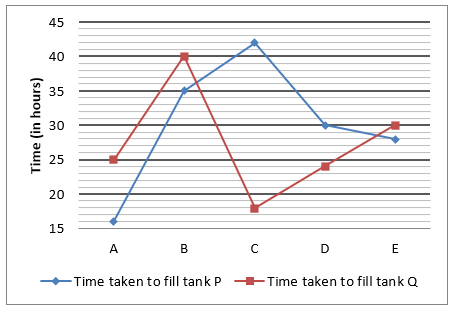Q1. Total capacity of tank – P is 360 liters more than total capacity of tank – Q. Pipe – B fills tank – Q in 33⅓ hours when it fills tank – Q with same efficiency with which it filled tank – P. Find total capacity of tank – P & Q together?
(a) 12840 liters
(b) 13620 liters
(c) 13180 liters
(d) 14760 liters
(e) 14340 liters

Q2. Pipe – A, C & E filled tank – Q in 29⅓ hours when they opened alternatively such that pipe – A fills in 1st hour, followed by pipe – C and then followed by pipe – E and pipe – C filled tank – Q with the efficiency with which it filled tank – P, then find total capacity of tank – P is what percent of that of tank – Q?
(a) 114⅓%
(b) 121⅓%
(c) 95⅔%
(d) Cannot be determined.
(e) None of the above.

Q3. Time taken by C & E together to fill tank – P is what percent of time taken by B & D together to fill tank – Q?
(a) 128%
(b) 112%
(c) 124%
(d) 120%
(e) 116%

Q4. Total capacity of tank – P is 3000 liters more than that of tank – Q. When pipe – B & D together filled tank – Q, then water supplied by pipe – D is 3375 liters. Find quantity of water supplied by pipe – A & E together in 6 hours in tank – P.
(a) 4950 liters
(b) 5450 liters
(c) 4250 liters
(d) 5950 liters
(e) 6650 liters

Q5. Water supplied by pipe – B, C & E together in tank – P in 5 hours is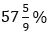of total capacity of tank – Q and pipe – A can fill 5040 liters in tank – Q in 14 hours. Find in how many hours pipe – D can fill 8232 liters in tank – P?
(a) 14 hours
(b) 16 hours
(c) 23 hours
(d) 17 hours
(e) 21 hours

Directions (6-10): Bar graph given below shows percentage of students passed out of total students in four different schools and percentage of girls passed out of total passed students in these four different schools in annual exam. Read the data carefully and answer the questions.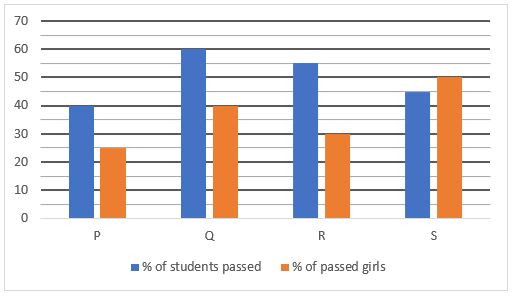Q6. Total boys passed from P is 900 and total girls failed from Q is 640. If total girls failed from Q is 36% less than of total students failed from Q, then find ratio of total students participated in exam from Q to that of from P?
(a) 5 : 9
(b) 5 : 7
(c) 3 : 5
(d) 5 : 6
(e) 4 : 7

Q7. Total boys failed from school Q is 60% of total students failed from that school and total boys passed from same school is 1440. Total failed boys from R is 192 more than total female passed from same school. Find difference between total girls failed from Q & R, if boys failed from R is 42% less than total students failed from R.
(a) 272
(b) 242
(c) 252
(d) 240
(e) 262

Q8. If total number of girls passed from S is 1125 and total failed boys are 133 ⅓% more than that of total failed girls from same school, then find difference between number of failed boys and failed girls from school S?
(a) 1100
(b) 1000
(c) 1110
(d) 900
(e) 1200

Q9. If difference between passed boys and passed girls from P is 600 and total boys passed from S is 1350 , then find total failed students from S is what percent more than total students participated from school P ?
(a) 15%
(b) 10%
(c) 12%
(d) 5%
(e) 20%

Q10. Total students participated in exam from school R is 50% more than that of from P and difference between passed boys from both the school is 2220, then find average number of girls passed from both the schools?
(a) 1100
(b) 1000
(c) 1390
(d) 900
(e) 1200

Directions (11-15): Study the bar chart given below and answer the following questions.
Bar chart shows the cost price of 5 different articles (A, B, C, D & E) and amount of discount allowed on these 5 articles.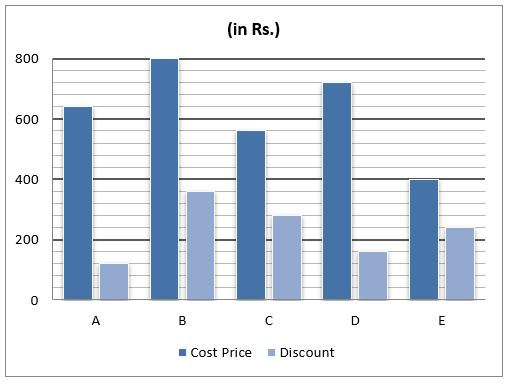Q11. Selling price of A is Rs.260 more than that of C and selling price of C is 50% more than discount allowed on C. Find marked price of A & C together is what percent of cost price of B & E together.

(a) 75%
(b) 125%
(c) 175%
(d) 100%
(e) 150%

Q12. D is marked 33⅓% above its cost price and amount of profit on B is 27.5% of marked price of B. If cost price of article – F is equal to selling price of article – B and selling price of article – F is 60% more than selling price of article – D, then find profit earned on article – F.
(a) Rs.150
(b) Rs.110
(c) Rs.70
(d) Rs.90
(e) Rs.40

Q13. Ratio of marked price of E to profit earned on E is 25 : 9 and marked price of C is equal to cost price of B. Find selling price of C & E together are how much more or less than cost price of A & B together?
(a) Rs.200
(b) Rs.120
(c) Rs.40
(d) Rs.160
(e) Rs.80

Q14. Marked price of A & B together is Rs.2000 and ratio of selling price of A to that of B is 17 : 21. Find amount of profit/loss earned by a person, if he sold 12 units of article – A and 17 units of article – B.
(a) Rs.1160
(b) Rs.1540
(c) Rs.1820
(d) Rs.820
(e) Rs.640

Q15. Profit earned on B is equal to that earned on E and selling price of B is equal to marked price of D. If selling price of D is 84% of its marked price, then find marked price of B & E together.
(a) Rs.2340
(b) Rs.2280
(c) Rs.2200
(d) Rs.2480
(e) Rs.2400

Solutions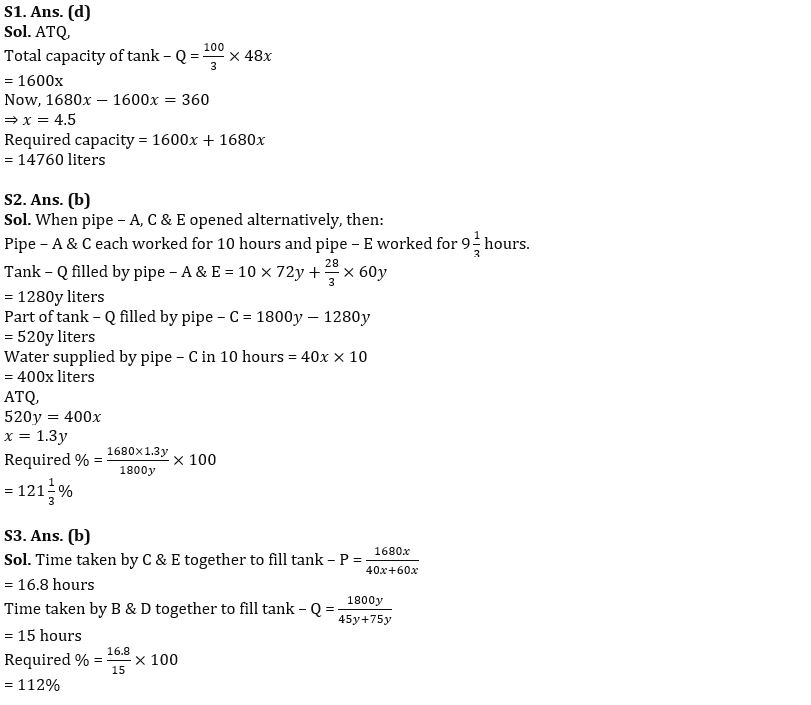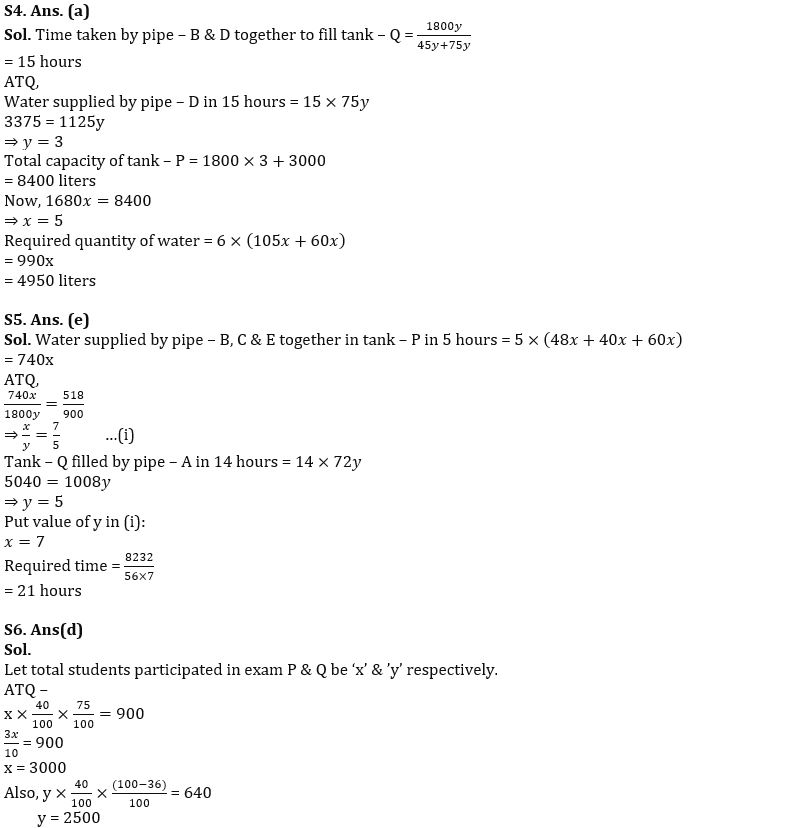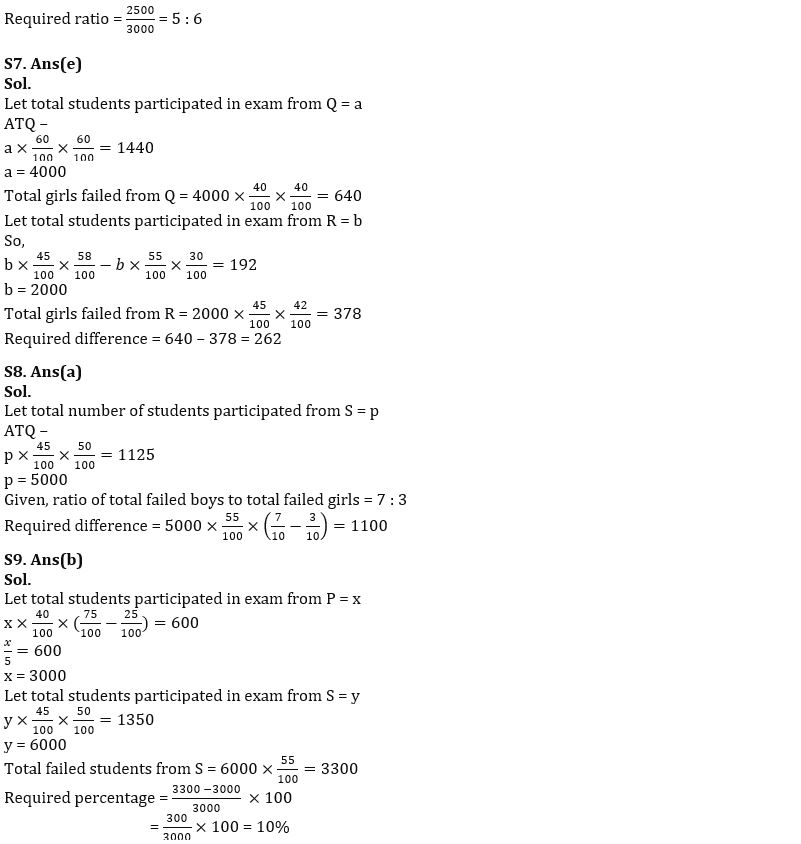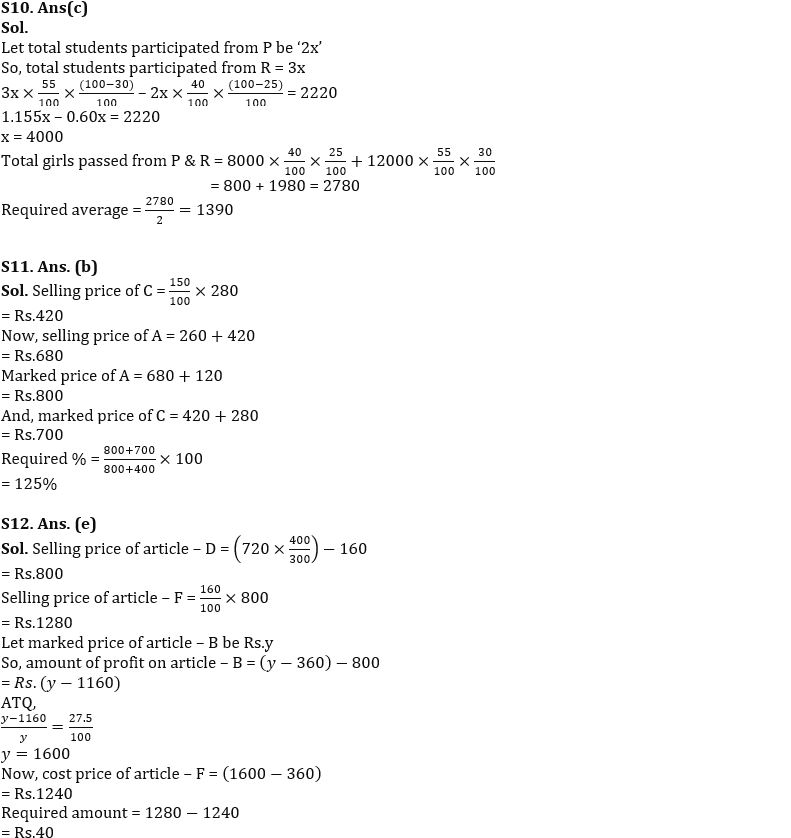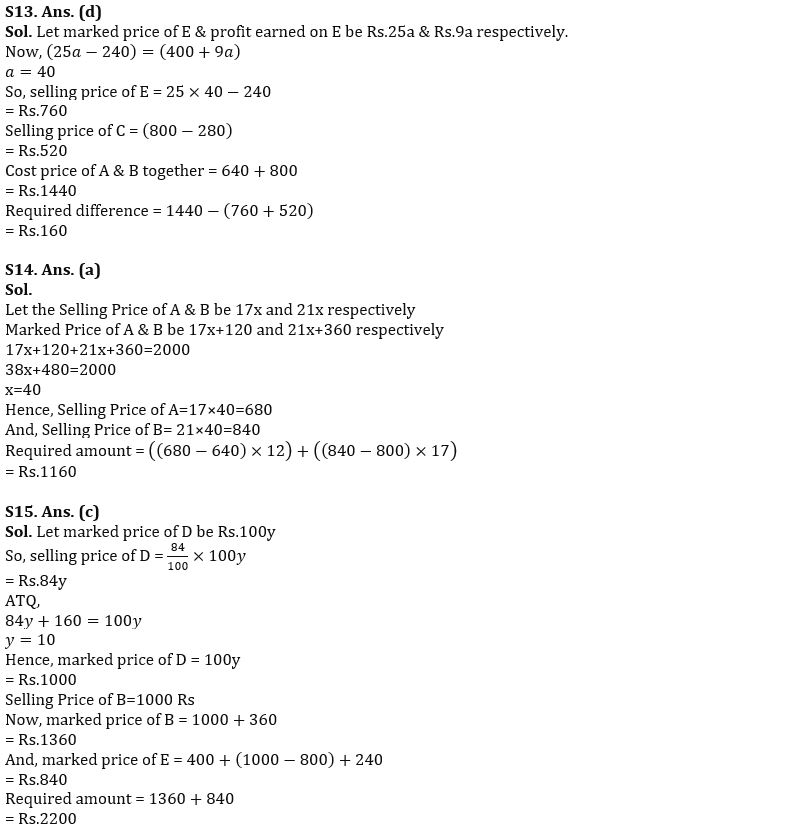## FAQs

### Q1. Total capacity of tank – P is 360 liters more than total capacity of tank – Q. Pipe – B fills tank – Q in 33⅓ hours when it fills tank – Q with same efficiency with which it filled tank – P. Find total capacity of tank – P & Q together? (a) 12840 liters (b) 13620 liters (c) 13180 liters (d) 14760 liters (e) 14340 liters

S1. Ans. (d)

#### Congratulations!